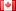# Revision history [back]

### Algorithm implementation in Sage

Input: Two undirected simple graphs G1 and G2, each having n vertices. Output: True if G1 ? = G2; False otherwise.

1 for i ? 1,2 do 2 Ai ? adjacency matrix of Gi 3 pi ? permutation equivalence class of Ai 4 A0 i ? lexicographically maximal element of pi 5 if A0 1 = A0 2 then 6 return True 7 return False

anyone have any idea how to implement this in Sage ?2 tried to improved stylefidbc2258 ●4 ●23 ●52

### Algorithm implementation in Sage

Input: Two undirected simple graphs G1 and G2, each having n vertices. Output: True True if G1 ? = G2; False False otherwise.

1

for i ? 1,2 do
2   Ai ? adjacency matrix of Gi
3   pi ? permutation equivalence class of Ai
4   A0 i ? lexicographically maximal element of pi
5 if A0 1 = A0 2 then
6   return True
7 return FalseFalse


anyone have any idea how to implement this in Sage ?3 retaggedFrédéricC3790 ●3 ●36 ●75

### Algorithm implementation in Sage

Input: Two undirected simple graphs G1 and G2, each having n vertices. Output: True if G1 ? = G2; False otherwise.

for i ? 1,2 do
Ai ? adjacency matrix of Gi
pi ? permutation equivalence class of Ai
A0 i ? lexicographically maximal element of pi
if A0 1 = A0 2 then
return True
return False


anyone have any idea how to implement this in Sage ?